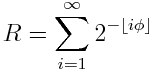# Golden strings and the rabbit constant

Golden strings are analogous to Fibonacci numbers, except one uses concatenation rather than addition.

Start with s1 = “1” and s2 = “10”. Then define sn = sn-1 + sn-2 where “+” means string concatenation.

The first few golden strings are

• “1”
• “10”
• “101”
• “10110”
• “10110101”

The length of sn is Fn+1, the n+1st Fibonacci number. Also, sn contains Fn 1’s and Fn-1 0’s. (Source: The Glorious Golden Ratio).

If we interpret the sn as the fractional part of a binary number, the sequence converges to the rabbit constant R = 0.7098034428612913…

It turns out that R is related to the golden ratio φ bywhere ⌊i φ⌋ is the largest integer no greater than iφ.

Here’s a little Python code to print out the first few golden strings and an approximation to the rabbit constant.

    from mpmath import mp, fraction

a = "1"
b = "10"
for i in range(10):
b, a = b+a, b
print(b)

n = len(b)
mp.dps = n
denom = 2**n
num = int(b, 2)

rabbit = fraction(num, denom)
print(rabbit)


Note that the code sets the number of decimal places, mp.dps, to the length of the string b. That’s because it takes up to n decimal places to exactly represent a rational number with denominator 2n.

## 4 thoughts on “Golden strings and the rabbit constant”

1. The rabbit constant calculation in the J language:

0.5#.|.0,;(;;{.)^:(6) 1;0
0.709803

written by Raul Miller @ forums.jsoftware.com